Warning

This documents an unmaintained version of NetworkX. Please upgrade to a maintained version and see the current NetworkX documentation.

# kl_connected_subgraph¶

kl_connected_subgraph(G, k, l, low_memory=False, same_as_graph=False)[source]

Returns the maximum locally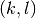-connected subgraph of G.

A graph is locally-connected if for each edge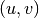in the graph there are at leastedge-disjoint paths of length at most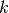joining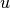to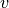.

Parameters: G (NetworkX graph) – The graph in which to find a maximum locally-connected subgraph. k (integer) – The maximum length of paths to consider. A higher number means a looser connectivity requirement. l (integer) – The number of edge-disjoint paths. A higher number means a stricter connectivity requirement. low_memory (bool) – If this is True, this function uses an algorithm that uses slightly more time but less memory. same_as_graph (bool) – If this is True then return a tuple of the form (H, is_same), where H is the maximum locally-connected subgraph and is_same is a Boolean representing whether G is locally-connected (and hence, whether H is simply a copy of the input graph G). If same_as_graph is True, then this function returns a two-tuple as described above. Otherwise, it returns only the maximum locally-connected subgraph. NetworkX graph or two-tuple

References